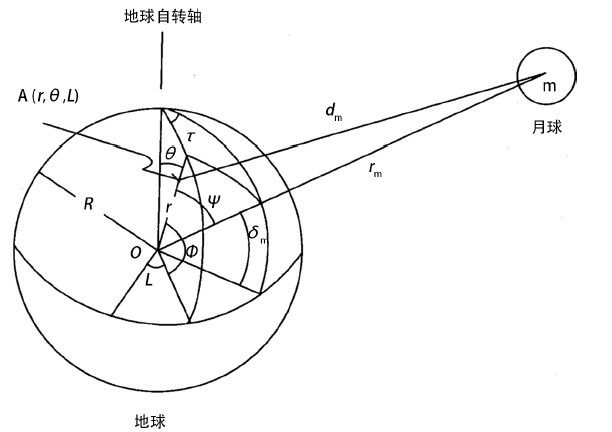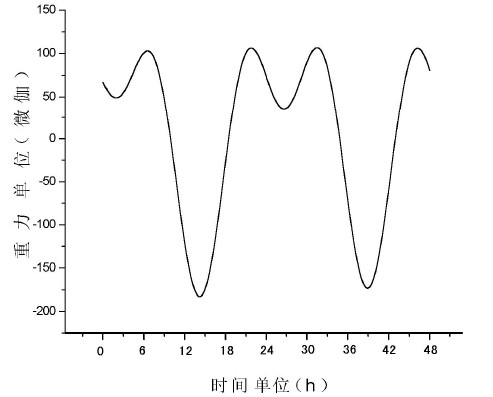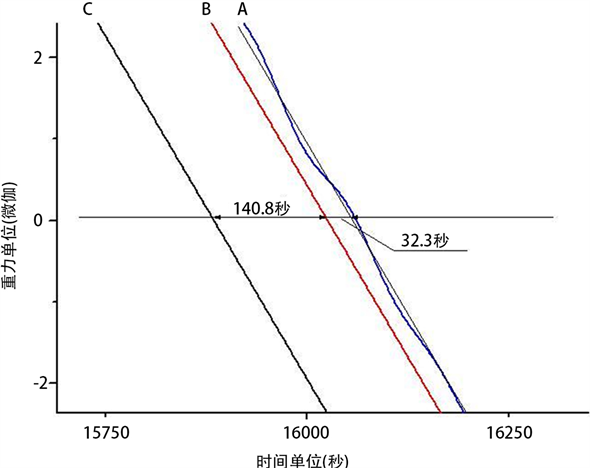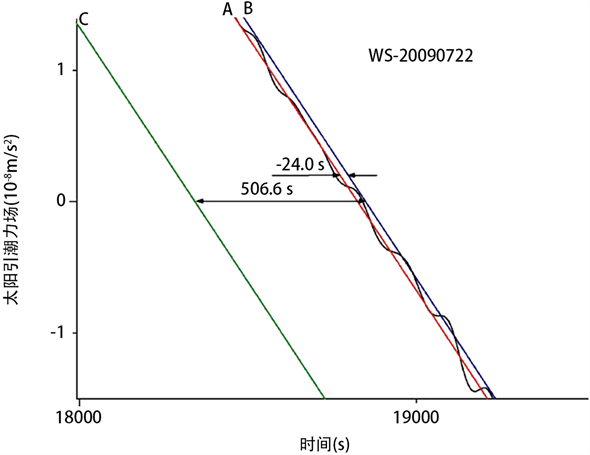# 固体潮观测确定引力相互作用传播速度与光速相同Solid Tide Observations Confirm That the Gravitational Interaction Speed Is the Same as the Speed of Light

• 全文下载: PDF(1668KB)    PP.47-57   DOI: 10.12677/AAS.2018.63004
• 下载量: 583  浏览量: 1,197

The law of universal gravity does not contain time. It has been regarded as a theory of action of distance by mainstream physics which means gravity can be transmitted instantaneously. The special theory of relativity indicates that the speed of light is the upper limit of all physical effects. Scientists have been looking forward to finding ways to measure gravitational speed. November 2012, “Science Bulletin” published “by the solid tide found gravity at the speed of light transmission of observation evidence”, reported that Chinese scientists with gravity solid tide observation data, in the time domain analysis, obtained the conclusion that gravitational propagation at the speed of light. In this paper, from the aspects of the theory of gravity tide, calculation, and determination of celestial body position, it is pointed out that the theory of earth tide implies that the speed of gravity is the same as the speed of light. Since the observed values of the earth tide agree with the theoretical values, it can be concluded that the velocity of the gravitational interaction is the same as the speed of light. According to the long sequence data of the superconducting gravimeter in Wuhan station, the phase difference between the moon M2 and the sun S2 with respect to the theoretical value is close to zero, which is consistent with the results of the earth tide theory. Again, the conclusion that gravitation is transmitted at the speed of light is obtained. The normal astronomical operation of correction of optical annual aberrations from the sun and the moon position indicates logically that the velocity of gravity interaction must be the same as the speed of light.

1. 引言

2. 重力固体潮理论值的计算表达

$F=-G\frac{Mm}{{r}_{m}^{2}}$ (1)Figure 1. Earth and moon coordinate position diagram. τ, δm, Ψ, rm, m, dm are the moon’s hour angle, declination, the polar angle of the moon, the distance from the moon’s heart to the center of the earth, the mass of the moon, and the distance from the moon’s heart to the point A. τs, δs, Ψs, rs, ms, ds are , respectively, The solar hour angle, declination, solar polar angle, the distance from the heliocentric to the geocentric, the mass of the sun, and the distance from the heliocentric to point A

$A\left(r,\theta ,L\right)$ 点的月球引力位 $V\left(A\right)$ 为   ：

$V\left(A\right)=-G\frac{m}{{d}_{m}}=-Gm\underset{0}{\overset{\infty }{\sum }}\frac{{r}^{n}}{{r}_{m}^{n+1}}{P}_{n}\left(\mathrm{cos}\psi \right)$ (2)

A点的引潮力位 $T\left(A\right)$ 等于天体对地球上单位质点的引力位 $V\left(A\right)$ 加上公转时单位质点的惯性离心力位 $Q\left(A\right)$

$T\left(A\right)=V\left(A\right)+Q\left(A\right)=-G\frac{m}{{r}_{m}}\underset{2}{\overset{\infty }{\sum }}{\left(\frac{r}{{r}_{m}}\right)}^{n}{P}_{n}\left(\mathrm{cos}\psi \right)$ (3)

$\Delta g=-\frac{\partial T}{\partial r}$ (4)Figure 2. Observations of gravity earth tide recorded on July 21-22, 2009 recorded by PET gravimeter of Wushi station, Xinjiang

3. 重力固体潮与日月引力的关系

4. 太阳视位置需作周年光行差校正到真位置

5. 重力固体潮理论包含了引力以光速传播假定

A测站t时刻重力固体潮理论值，太阳位置要用经光行差校正后的真位置 ${X}_{S}^{R}\left(t-\Delta {t}_{s}\right)$ 的时空值，太阳引力从 $\left(t-\Delta {t}_{s}\right)$ 时刻发出，t时刻到观测点A。

5.1. 对重力固体潮观测数据在时间域中的分析Figure 3. A comparison of three curves of observational solid tides with theoretical values of solid tides and momentary theoretical values of gravitational force on July 22, 2009 in Wushi Station, XinjiangFigure 4. An enlarged view of the partial enlargement box in Fig3 of the observational curve of the earth tide in (A), the theoretical curve of the solid tide (B) and the theoretical curve (C) of the instantaneous propagation of the gravitation. The three curves are roughly parallel. If the observation value is based, the theoretical curve of the solid tide is about 32 seconds ahead of time, and the of the instantaneous propagation of gravity is about 141 seconds ahead of the observational curveFigure 5. On July 22, 2009, Wushi, Xinjiang, compared the three curves of the daily tide observation curve (A) with the daily tide theoretical value (B) and the instantaneous propagation of the tide’s theoretical value (C) 

5.2. 对重力固体潮长序列观测数据在频率域中的分析Table 1. The comparison of tidal parameters before and after tidal load correction in Wuhan station 

6. 光行差校正操作，逻辑上隐含：引力速度只能与光速相同

7. 结论

2017年10月，LIGO和室女座探测器在伽玛射线卫星和光学望远镜两秒钟内接收到来自同一方向的引力波信号。这证实了引力波的速度与光速相同。

  Einstein, A. (1954) The Meaning of Relativity. 5th Edition, Princeton University Press, Princeton, NJ.  Tang, K.Y., Hua, C., Wen, W., et al. (2013) Observational Evidences for the Speed of the Gravity Based on the Earth Tide. Chinese Science Bulletin, 58, 4-5. https://doi.org/10.1007/s11434-012-5603-3  汤克云, 华昌才, 文武, 等. 从地球重力潮观测发现引力以光速传播的证据[J]. 科学通报, 2013(58): 907-911  https://en.wikipedia.org/wiki/Gravity  Kopeikin, S.M. and Fomalont, E.B. (2002) General Relativistic Model for Experimental Measurement of the Speed of Propagation of Gravity by VLBI. Astrophysical Journal, 398, L5-L8.  P. 梅尔基奥尔. 行星地球的固体潮[M]. 北京: 科学出版社, 1984.  许厚泽, 等. 固体地球潮汐[M]. 武汉: 湖北科学技术出版社, 2010: 2-4.  Ray, R.D., Eanes, R.J. and Chao, B.F. (1996) Detection of Tidal Dissipation in the Solid Earth by Satellite Tracking and Altimetry. Nature, 381, 595-597. https://doi.org/10.1038/381595a0  Ray, R.D., Eanes, R.J. and Lemoine, F.G. (2001) Constraints on Energy Dissipation in the Earth’s Body Tide from Satellite Tracking and Altimetry. Geophysical Journal International, 144, 471-480. https://doi.org/10.1046/j.1365-246x.2001.00356.x  孙和平, 朱耀仲, 主编. 大地测量与地球动力学进展[M]. 武汉: 湖北科学技术出版社, 2004: 421.  刘琳霞, 邵成刚. 广义相对论中引力传播速度的测量问题[J]. 科学通报, 2014, 59(4-5): 323-327.  Laplace, P.S. (1966) Mecanique Celeste. Chelsea Publication, New York.  Flandern, T.V. (1998) The Speed of Gravity—What the Experiments Say. Physics Letters A, 250, 1-11. https://doi.org/10.1016/S0375-9601(98)00650-1